# ISEE Lower Level Quantitative : How to find the probability of an outcome

## Example Questions

1 2 3 5 Next →

### Example Question #41 : Outcomes

A store has a bin of books on sale. In the bin, there arenovels,comic books,reference books, andpicture books. What is the probability that someone will choose a novel from the bin?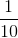Explanation:

Probability can be expressed as a fraction. The numerator represents the total number of what is being chosen and the denominator represents the total number of items that can be chosen. In this problem, there arenovels and a total ofbooks. This is represented as the fraction: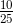. This can be reduced to. The answer is.

### Example Question #1601 : Isee Lower Level (Grades 5 6) Quantitative Reasoning

There aremarbles in a bag:are blue,are white,are yellow, andare green. What is the probability of choosing blue or green at random?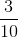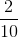Explanation:

Probability can be expressed as a fraction. The numerator represents the total number of what is being chosen and the denominator represents the total number of items that can be chosen. In this problem, there areblue and green marbles and a total ofchocolates. This is represented as the fraction: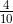. This can be reduced to. The answer is.

### Example Question #42 : Outcomes

A bag containsred socks andpurple socks. What is the chance that I pick a purple sock from the bag?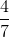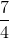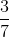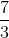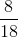Explanation:

To find the probability of picking a purple sock from the bag of socks, we need to set up a fraction like this: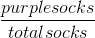. The problem tells us that we havepurple socks, so we can put that on the top of the fraction. The total number of socks is equal topurple socks +red socks, giving us a sum of(which goes on the bottom of the fraction). That gives us achance of picking a purple sock from the bag!

### Example Question #41 : Data Analysis And Probability

Mr. Brown hasred shirts,blue shirts,yellow shirts, andgreen shirts. If he selects a shirt randomly, what color shirt has ainchance of being chosen?

Red

Yellow

Green

Blue

Green

Explanation:

Recall what a probability is: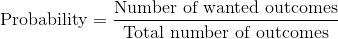Now, we have a total number ofoutcomes because that is the number of shirts Mr. Brown has to choose from.

We can then set up the following ratio: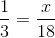Notice that you multiplybyto get. The value forthen should be. Out of all the shirts Mr. Brown has to choose from, the only color shirt that he hasof is green.

### Example Question #42 : Data Analysis And Probability

There is a raffle for a basket of cookies. Bill buys 3 tickets, Julie buys 2 tickets, Rob buys 4 tickets, and 5 other people buy 2 tickets each. What are the chances that Rob will win the raffle?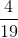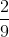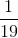Explanation:

If Bill buys 3 tickets, Julie buys 2 tickets, Rob buys 4 tickets, and 5 other people buy 2 tickets each, there are a total of 19 tickets: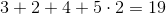Thus, the chance of Rob winning is.

### Example Question #41 : How To Find The Probability Of An Outcome

Ebony has a drawer of socks with five different colors: green, purple, black, white, and yellow. The probability of her choosing a white sock is 3 out of 7. Which combination of socks is possible?

3 white socks and 7 other socks

6 white socks and 14 other socks

7 white socks and 21 other socks

9 white socks and 12 other socks

1 white sock and 4 other socks

6 white socks and 14 other socks

Explanation:

We are looking at probability and proportion in this problem.

If there is a 3 out of 7 chance of getting a white sock, this can be expressed asor 3:7.

Then, we must find an equivalent combination, which would be 6:14.

### Example Question #43 : Data Analysis And Probability

There are 64 students in a science class. If there are 24 boys, what is the ratio of the number of girls to the total number of students in the science class?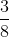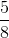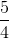Explanation:

We are given the ratio of boys in the class, which is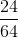.

First, we must reduce that fraction by finding the greatest common denominator, which is 8.

The fraction is then reduced to.

We are looking for the ratio of girls in the gym class.

So we subtract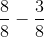This leaves us with our answer of### Example Question #44 : Data Analysis And Probability

Mary has a bag withcookies.cookies are chocolate chip,cookies are sugar, andcookies are oatmeal raisin. What is the chance that Mary randomly selects a sugar cookie from the bag?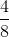Explanation:

To find the probability of Mary picking a sugar cookie from the bag of cookies, we need to set up a fraction like this: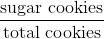.

The problem tells us that Mary hassugar cookies, so we can put that on the top of the fraction. The problem also tells us that Mary hastotal cookies, so we can put that on the bottom of the fraction. That gives Mary a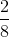chance of picking a sugar cookie.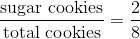Sinceis not an answer choice, we need to reduce the fraction. To reduce a fraction means to divide the top (numerator) and the bottom (denominator) by a common factor that both numbers share. The numbersandboth have a common factor of, so we can divide the top and the bottom byto get the correct answer of.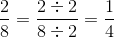1 2 3 5 Next →

### All ISEE Lower Level Quantitative Resources